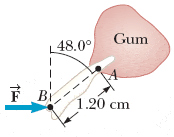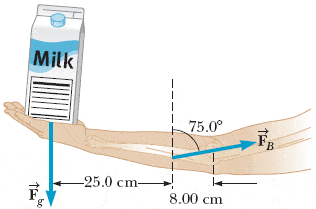# How do I find the torque about point A? My answer differs significant from the correc

A dental bracket exerts a horizontal force of 83.1 N on a tooth at point B in the figure. What is the torque on the root of the tooth about point A?_______ N · m counterclockwise

I just did:

τA = [ (83.1 N)sin 48 ](1.20 cm) = 74.1

Your response differs significantly from the correct answer. Rework your solution from the beginning and check each step carefully.

## Answers and Replies

The distance is given in cm. You have to use m to get energy in J.
Also, I think the angle between the 2 vectors is 90°+48.0°

The distance is given in cm. You have to use m to get energy in J.
Also, I think the angle between the 2 vectors is 90°+48.0°

Ohh ok...
thanks!

What about this one?

A cook holds a 1.13-kg carton of milk at arm's length (see the figure below). What force vector F =B must be exerted by the biceps muscle? (Ignore the weight of the forearm. State the magnitude of the force vector FB.)I tried doing...

1) Fg = ma
Fg = (1,13 kg)(9.81)
Fg = 11.08

2) Ta = (11.08 N)(0.25 m) = 3.997

3) Tb = (FB cos 75)(0.08)
4) Set Ta = Tb

3.997 = (FB cos 75)(0.08)
133 N = FB

INCORRECT: Your response differs from the correct answer by more than 10%. Double check your calculations.

For the carton, the rotation is about the bone joint which is 33cm from the weight vector, not 25cm.

For the torque created by the muscle, draw out the r and F vectors and determine the angle between them from your drawing.

For the carton, the rotation is about the bone joint which is 33cm from the weight vector, not 25cm.

For the torque created by the muscle, draw out the r and F vectors and determine the angle between them from your drawing.

Ok this is what I did after looking it up online...

1) Determine the horizontal and vetical components of the force exerted by the biceps muscle where you see an angle - make a triangle to determine which side is cos and which side is sin.

Horizontal component
(FB)x = FBsin 75

Vertical component
(FB)y = FBcos 75

2) I googled this but I dont understand the "determining axis of rotation" part...

ƩT: -Fg(25 cm + 8 cm) - (FB)y(8 cm) = 0

-(1.13)(-9.81)(33 cm) - FBcos 75(8 cm) = 0
365.8 - FBcos 75(8 cm) = 0
365.8 = FBcos 75(8 cm)
45.7 = FBcos 75
176.7 N = FB

I don't understand how they got the idea to add [F x r of the WHOLE ARM] MINUS (FB)y(r of PART of the arm)

When the forearm moves, it rotates about the elbow joint - this is the axis of rotation, (imagine a line through the elbow perpendicular to the diagram).
To calculate the torque, you need the force, and the distance of this force from the axis of rotation.

For the carton, the weight vector is 33cm from this axis.
For the biceps muscle, the force in the diagram is 8cm from this axis.

When the forearm moves, it rotates about the elbow joint - this is the axis of rotation, (imagine a line through the elbow perpendicular to the diagram).
To calculate the torque, you need the force, and the distance of this force from the axis of rotation.

For the carton, the weight vector is 33cm from this axis.
For the biceps muscle, the force in the diagram is 8cm from this axis.

Ohhh ok that makes perfect sense!

So whenever Im dealing with a Torque problem, I ALWAYS have to determine my main axis of rotation??

Yes, this is the first thing you should determine because you need to have the distance from the axis of rotation to the point where the force is being applied.

Yes, this is the first thing you should determine because you need to have the distance from the axis of rotation to the point where the force is being applied.

Ok thanks!!